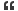1. ## Prisoners problem....

Here is one of the famous challenging puzzle...

There are 100 prisoners assigned by numbers in 1 to 100 . Any number can be assigned to them . They need not be unique. They can talk one time before they assigned and then don't have any connection. Each one is requested to guess his number (they can use different strategies). He can see their numbers (but not their guess).
How can they do it so at least one of them guesses correctly his number?Reply With Quote

2. ## Re: Prisoners problem....

Hi James,

I think if you assigned the unique number for prisoner then only we have a solution. Otherwise according to my knowledge there is no general solution for this problem...

If you have a solution then post here...i will find the error.

-------------------
sureshReply With Quote

3. ## Re: Prisoners problem....

I have the solution for this problem. Let us wait for few more days so that others can give a try.

Definitely you will be having the solution in this thread by the next week end. It can be either from me or from some one elseReply With Quote

4. ## Re: Prisoners problem....

Hi James,

We are all waiting for the solution for this problem....Almost 10 days over...

--------------------
sureshReply With Quote

5. ## Re: Prisoners problem....

Sorry for the delay to post my solution.

Let us assume that the sum of all number assigned to 100 prisoners is S(P) and the number assigned to the first prisoner is n1, the number to the second prisoner is n2 and so on.

That is, S(P) = n1 +n2+n3 +...+n100.

And it is obvious that Mod(S(P),100) ranges between 0-99.

Here is my strategy that must work in all cases,

The first prisoner always assumes that "Mod(S(P),100)" is going to be 0
The second prisoner assumes that "Mod(S(P),100)" is going to be 1
so on...

It is certain that exactly one of the 100 prisoners is right with his guess.

Now, The first prisoner in his turn
1. Add all numbers assigned to rest of the people (n2+n3+...+n100). Let us say the value is S(p1).
2. Find "Mod(s(p1),100)". Let us say he has got the number np1.
3. Find 100 -np1, which is going to be his guess. Since he guess that "Mod(S(P),100)" is going to be 0

Let be brief this with an example.

Let use assume that he has got 5078 at the end of first step

Step2 mod(5078,100) = 78
Step3 100 -78 = 22
Hence the guess will be made by the first prisoner is 22.

Now, The second prisoner
1. Add all numbers assigned to rest of the people (n1+n3+...+n100). Let us say the value is S(p2).
2. Find "Mod(s(p2),100)". Let us say he has got the number np2.
3. Find 100+1 -np2, which is going to be his guess.

Example if the second person got 5078 at the end of first step then his guess would be 100 + 1 -78 = 23.

All other 99 prisoners also will make their guess as described above. It is obvious that one their guess on value of "Mod(S(P),100)" must be right. Hence that prisoner's guessing on the number assigned him will also be right.Reply With Quote

6. ## Re: Prisoners problem....

Hi James,

For example if i assigned the numbers for first prisoner is 90 and other prisoners for 2.

According to your solution First prisoner guess is (198 mod 100) = 98
Next 100-98 = 2 => wrong

Second prisoner guess is (286 mod 100) = 86
Next 100+1 - 86 = 15

Third prisoner guess is (286 mod 100) = 86
Next 100+2 - 86 = 16
.
.
.
so on...

Here which prisoner guess goes right? If i am wrong then correct me...

-----------------------
sureshReply With Quote

7. ## Re: Prisoners problem....

let us take 89th prisoner . He guesses that the reminder is going to be 88

Hence his guess would be 100+88 -86 =102 (mod 100) = 2Reply With Quote

8. ## Re: Prisoners problem....

James bhai, tera ye sawaal ekdam vague hai. I believe that the only person who can guess his number correctly is 100th.

You have tried to apply your maths well, but it does not seem to work. At least for me.Reply With Quote

9. ## Re: Prisoners problem....

Have every Prisoner guess the same number!Reply With Quote

10. ## Re: Prisoners problem....Originally Posted by richardpaulhallHave every Prisoner guess the same number!
It is not correct.

if everyone guesses say 3 and what if nobody is assigned 3 ???Reply With Quote

11. ## Re: Prisoners problem....

perhaps you did not pay much attention to my solution...just kiddingReply With Quote

####Posting Permissions

• You may not post new threads
• You may not post replies
• You may not post attachments
• You may not edit your posts
•